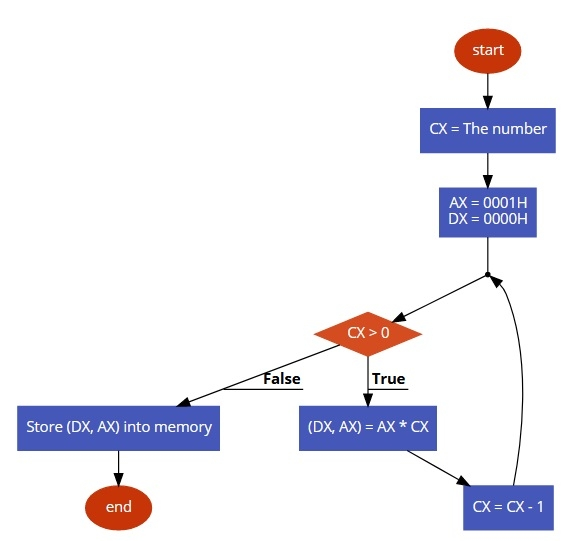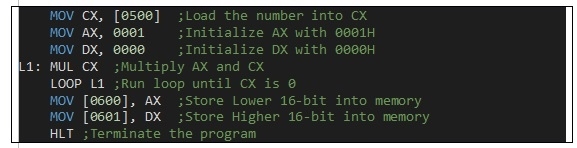# 8086 program to find the factorial of a number

In this program we will see how to find the factorial of a number.

## Problem Statement

Write 8086 Assembly language program to find the factorial of a number stored in memory offset 500. Store the result at 600 and 601 memory offset.

## Discussion

To find the factorial of a number n we have to repeatedly multiply the numbers from 1 to n. We can do the same by multiplying the number and decrease it until it reaches 1. So the sequence will be

In this program we are taking the number into counter register then decrease it and multiply, If the result exceeds the range of 16-bit, the 32-bit result can be found in register DX and AX. After that we are storing the result into memory offset 600 and 601.

Data

500
06

## Flow Diagram## Program## Output

Data

600
D0
601
02

Updated on: 30-Jul-2019

9K+ Views